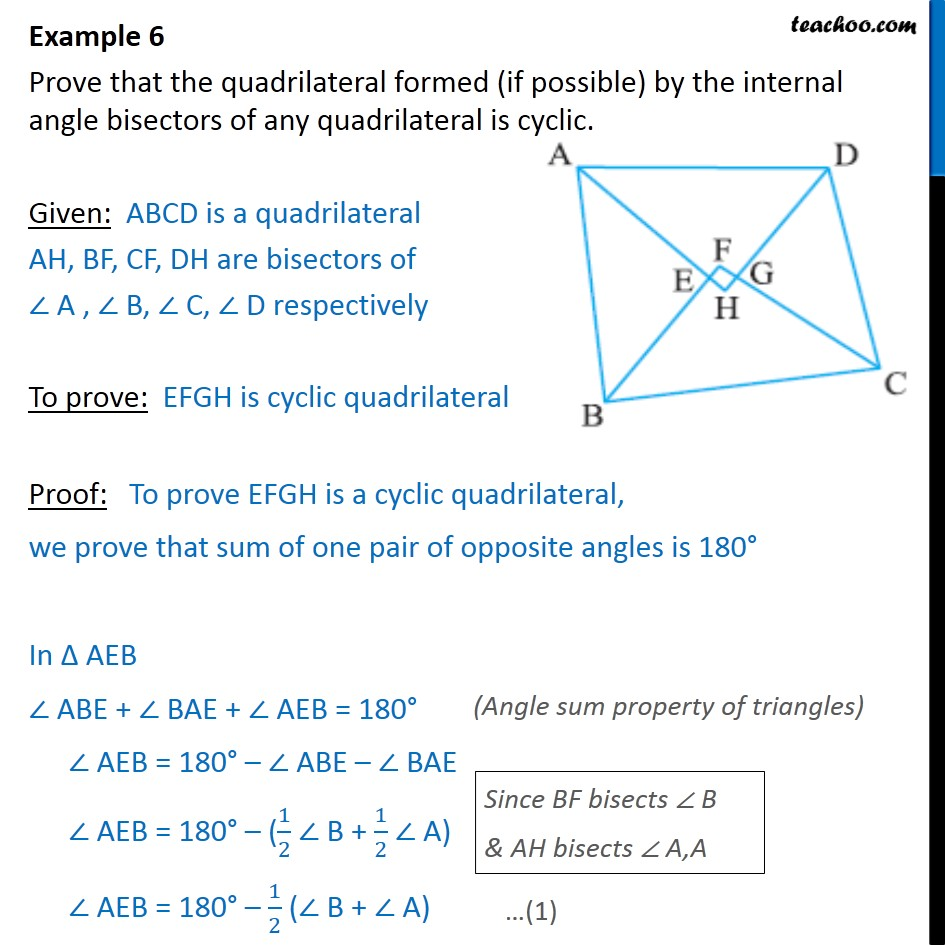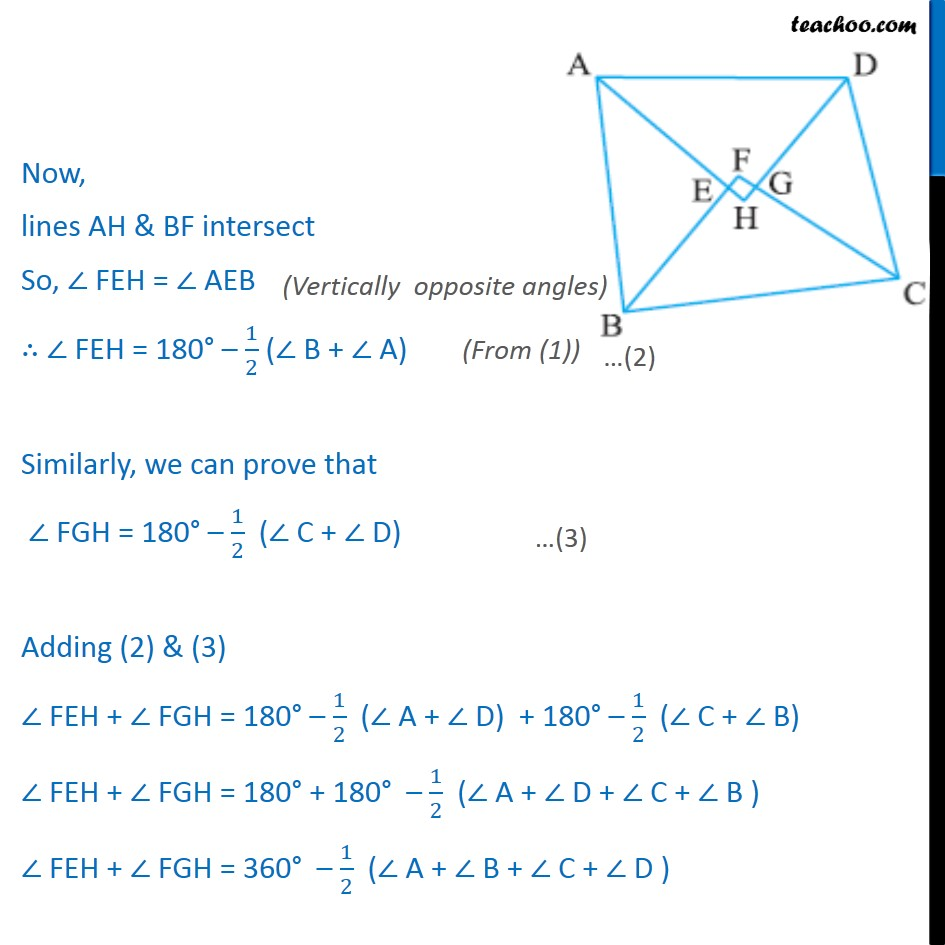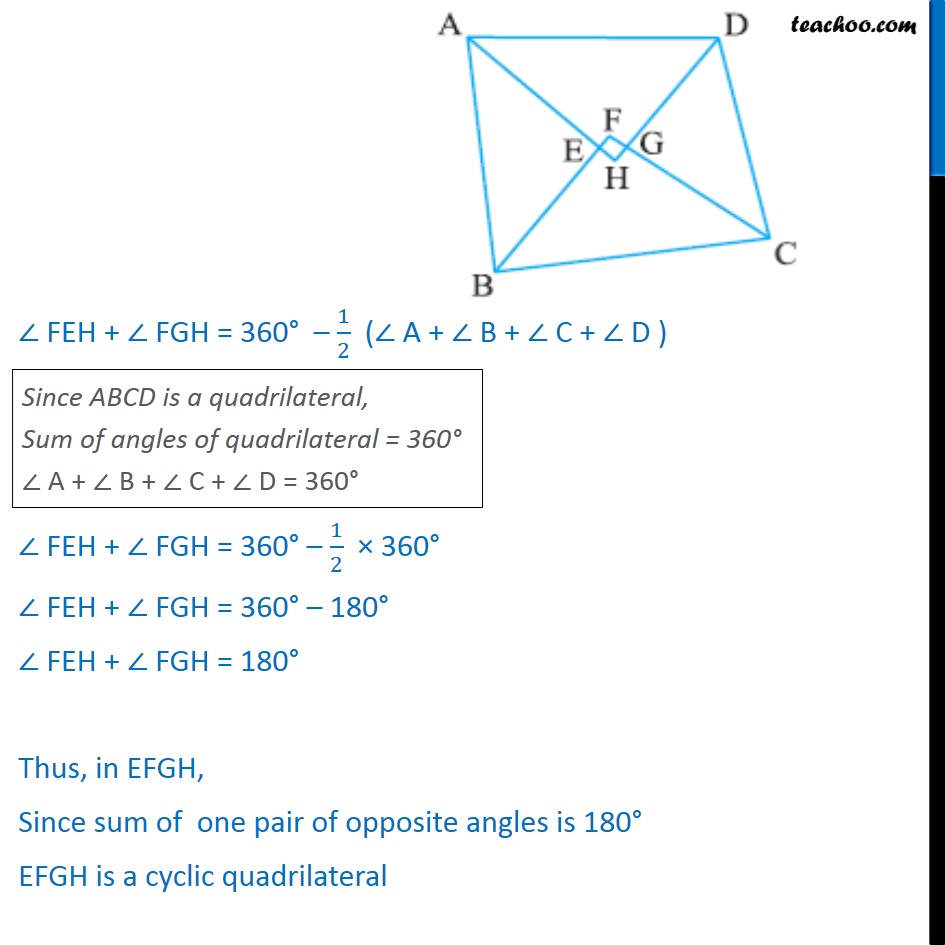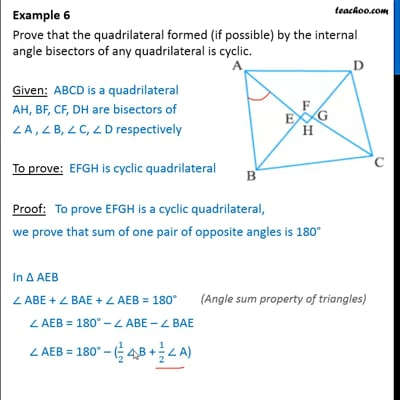Examples

Chapter 10 Class 9 Circles
Serial order wiseThis video is only available for Teachoo black users

Get live Maths 1-on-1 Classs - Class 6 to 12

### Transcript

Example 6 Prove that the quadrilateral formed (if possible) by the internal angle bisectors of any quadrilateral is cyclic. Given: ABCD is a quadrilateral AH, BF, CF, DH are bisectors of A , B, C, D respectively To prove: EFGH is cyclic quadrilateral Proof: To prove EFGH is a cyclic quadrilateral, we prove that sum of one pair of opposite angles is 180 In AEB ABE + BAE + AEB = 180 AEB = 180 ABE BAE AEB = 180 (1/2 B + 1/2 A) AEB = 180 1/2 ( B + A) Now, lines AH & BF intersect So, FEH = AEB FEH = 180 1/2 ( B + A) Similarly, we can prove that FGH = 180 1/2 ( C + D) Adding (2) & (3) FEH + FGH = 180 1/2 ( A + D) + 180 1/2 ( C + B) FEH + FGH = 180 + 180 1/2 ( A + D + C + B ) FEH + FGH = 360 1/2 ( A + B + C + D ) FEH + FGH = 360 1/2 ( A + B + C + D ) FEH + FGH = 360 1/2 360 FEH + FGH = 360 180 FEH + FGH = 180 Thus, in EFGH, Since sum of one pair of opposite angles is 180 EFGH is a cyclic quadrilateral